• 多项式方程计算器
2022-03-07 10:28:07

写的很菜---望大佬指点（或者轻喷

题目如下：

标题：
一元稀疏多项式计算器
类别：
综合
时间限制
2S
内存限制
1000Kb
问题描述
一元n次多项式𝑝0𝑋𝑒0+𝑝1𝑋𝑒1+⋯+𝑝𝑖𝑋𝑒𝑖+⋯+𝑝𝑛𝑋𝑒𝑛 项数较少时成为一元稀疏多项式，例如：3+6𝑋3−2𝑋8+12𝑋20是一个一元稀疏多项式。设计一个一元稀疏多项式计算器程序完成两个一元稀疏多项式的加减法，输出结果多项式的各项系数和指数。
输入说明
输入数据第1行为3个正整数n，m，t。其中 n表示第一个多项式的项数，m表示第二个多项式的项数，t表示运算类型，0为加法，1为减法。数据的第2行包含2n个整数，每两个整数分别表示第一个多项式每一项的系数和指数；第3行包含2m个整数，每两个整数分别表示第二个多项式每一项的系数和指数。两个多项式的每项是按照指数递增的形式给出的，例如对于多项式3+6𝑋3−2𝑋8+12𝑋20，对应的输入为3 0 6 3 -2 8 12 20
输出说明
运算结果按指数从低到高的顺序在以多项式形式（见输出样例）输出结果，注意系数为负数时输出减号，系数为0时不输出该项，指数为1时不输出指数。
输入样例
6 2 0
1 0 1 1 1 2 1 3 1 4 1 5
-1 3 -1 4
输出样例
1+x+x^2+x^5

虽然整体的思路并不难想，但是若是在考试环境下想出输出时候的各种可能的情况，想必这回成为一道经典题目的（（（

#include <stdio.h>
int main(void)
{
int n,m,t;
scanf("%d %d %d",&n,&m,&t);
int a1;
int a2;
int b1;
int b2;
for(int i = 0;i<n;i++){
scanf("%d %d",&a1[i],&a2[i]);
}
for(int i = 0;i<m;i++){
scanf("%d %d",&b1[i],&b2[i]);
}
for(int i = 0;i<n;i++){
for(int j = 0;j<m;j++){
if(a2[i] == b2[j]){
if(t) a1[i] -= b1[j];
else  a1[i] += b1[j];
b1[j] = 0;
b2[j] = 0;
}
}
}
int k = 0;
for(int i = 0;i<m;i++){
if(b1[i]!=0){
a1[n+k] = b1[i];
a2[n+k] = b2[i];
k++;
}
}
n = n+k;
for(int i = 0;i<n;i++){
if(a1[i] == 0){
for(k = i;k<n-1;k++){
a1[k] = a1[k+1];
a2[k] = a2[k+1];
}
n--;//这里是比较核心和关键的一个地方
i--;
}
}
for(int i = 0;i<n-1;i++){
for(int j = 0;j<n-1-i;j++){
if(a2[j] > a2[j+1]){
int temp = a2[j];
a2[j] = a2[j+1];
a2[j+1] = temp;
temp = a1[j];
a1[j] = a1[j+1];
a1[j+1] = temp;
}
}
}
if(a1 != 1) printf("%d",a1);
if(a2 == 0) {if(a1 == 1) printf("1");}
else if(a2 == 1)printf("x");
else printf("x^%d",a2);
for(int i = 1;i<n;i++){
if(a1[i] > 0) printf("+");
else if(a1[i] == -1) printf("-");
if(a1[i]!=1 && a1[i]!=-1) printf("%d",a1[i]);
if(a2[i] == 0);
else if(a2[i] == 1)printf("x");
else printf("x^%d",a2[i]);
}
return 0;
}算法 动态规划 c++ c语言
更多相关内容
• 用二叉树计算多项式，包含两个多项式之间的加减乘除幂运算和合并常数，以及对单个多项式的x赋值并求值。还另外用MFC写了程序界面，使得计算器操作简化。运行环境：Visual Studio 2019
• 高次方程计算，以分数格式输出答案。后期空闲再加入多元一次方程组进去。
• 支持多项式方程以及含有指数，对数和三角函数的方程。方程计算器可以找到方程的数值解和准确解。方程的解是简化的，所以它可能与你所期望的形式不同。支持复杂的解和复杂的方程组,支持复变函数。方程计算器如何在线...

方程求解给定变量的方程组。支持多项式方程以及含有指数，对数和三角函数的方程。方程计算器可以找到方程的数值解和准确解。方程的解是简化的，所以它可能与你所期望的形式不同。支持复杂的解和复杂的方程组,支持复变函数。

方程计算器如何在线使用

通常我们买的学生用计算器是不能直接计算一元二次方程，一元三次方程的，但是在网上我们却能方便准确的计算出来，而且不用下载就可直接使用的哦…

对于一元一次，一元二次方程，我们可以进入此网址http://www.jiefangcheng.net/，或者输入“在线解方程”即可，界面如下，下侧有使用须知，很简单方便，下面举个简单例子介绍怎么使用。在框里输入2x+8=20，点击“点我解方程”，计算步骤和结果就在下方显示了,同样输入2X^2+8=26，得出结果是±3。注意“^”是英文状态下按shift+6打出来的。

当然也可以输入2 x^2+x+8 = 18带两个X比较复杂点的式子，总之是很方便使用的。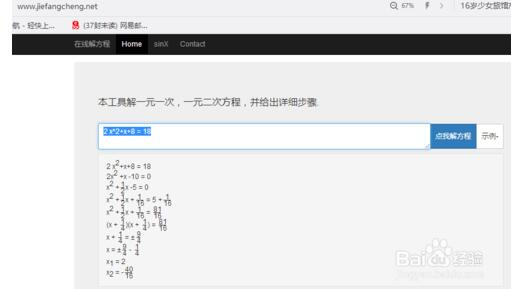类似这样的在线计算一元一次，一元二次方程的网站有很多，如：计算器在线网，这里你不用输入x，只需要在前边输入系数即可，相比来说这种方法更简单。那么我们看到这两个工具的计算结果是一样的。下边再介绍个复杂一些的一元三次方程的计算，叫九九参考计算网，网址如下：http://www.99cankao.com/algebra/cubic-equation.php，界面如下，方法和上一个的基本一样。下边我们来举个例子，我们在4个空白框里分别输入1，看到结果如下，很神奇吧！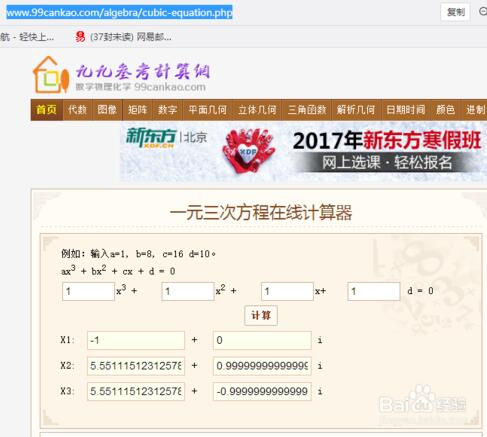其实学生用的计算器的计算也可以在网上在线计算的，网址如下：http://www.jisuanqinet.com/shuxue/bangbang.html，界面简单清晰，相信您也会喜欢的！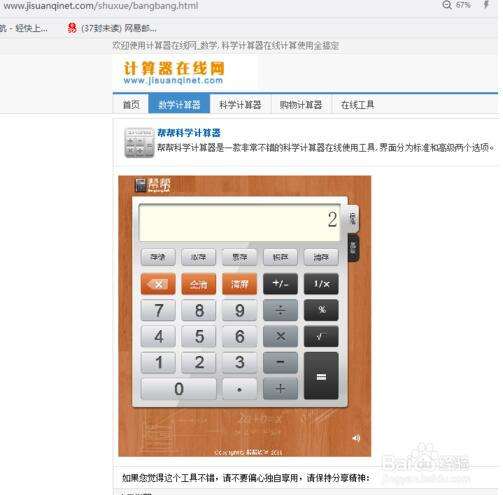展开全文• /**完成多项式计算器，可以进行多项式的加法，减法，乘法三种运算。*/import java.util.ArrayList;import java.util.List;import java.util.Scanner;import java.util.Stack;public class ExpressionMain {public ...

/**

完成多项式的计算器，可以进行多项式的加法，减法，乘法三种运算。

*/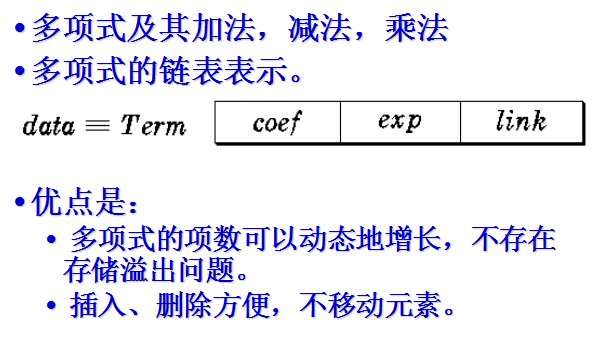import java.util.ArrayList;

import java.util.List;

import java.util.Scanner;

import java.util.Stack;

public class ExpressionMain {

public static void main(String[] args) {

expressionCalculator();

}

private static void expressionCalculator() {

System.out.println("This is a polynomials program.\n" +

"Please enter a valid command:\n" +

"[=] Return a Polynomial.\n" +

"[+] Sum two Polynomial.\n" +

"[-] Difference two Polynomial。 \n" +

"[*] Mult one Polynomial with a term Polynomial.\n" +

"[Q] Quit.\n");

Scanner scanner = new Scanner(System.in);

boolean isTheWorldAlive = true;

Stack stack = new Stack();

while (isTheWorldAlive) {

System.out.println("Select command and presss :");

String command = scanner.next();

if ("?".equals(command)) {

boolean isCurrentExpressionInProgress = true;

Term current = new Term();

boolean firstTime = true;

while (isCurrentExpressionInProgress) {

System.out.println("coefficient?");

int xishu = scanner.nextInt();

if (xishu != 0) {

System.out.println("exponent?");

int zhishu = scanner.nextInt();

if (zhishu != 0) {

if(firstTime){

current.setXishu(xishu);

current.setZhishu(zhishu);

firstTime = false;

}else{

Term temp = current;

while(temp.next != null){

temp = temp.next;

}

temp.next = new Term(xishu, zhishu);

}

} else {

System.out.println("The following has been pushed to Stack:");

System.out.println(current.toString());

stack.push(current);

isCurrentExpressionInProgress = false;

}

} else {

System.out.println("The following has been pushed to Stack:");

System.out.println(current.toString());

stack.push(current);

isCurrentExpressionInProgress = false;

}

}

} else if("+".equals(command)){

}else if("-".equals(command)){

System.out.println(Expression.minus(stack.pop(), stack.pop()));

}else if("*".equals(command)){

System.out.println(Expression.mutiple(stack.pop(), stack.pop()));

}

else if ("Q".equalsIgnoreCase(command)) {

isTheWorldAlive = false;

}

}

}

}

class Term {

private int xishu;

private int zhishu;

Term next = null;

Term(){}

Term(int xishu, int zhishu){

this.xishu = xishu;

this.zhishu = zhishu;

this.next = null;

}

public int getXishu() {

return xishu;

}

public void setXishu(int xishu) {

this.xishu = xishu;

}

public int getZhishu() {

return zhishu;

}

public void setZhishu(int zhishu) {

this.zhishu = zhishu;

}

public String toString(){

String toString = xishu+"X^"+zhishu;

Term term = this.next;

while (term != null){

int nextXishu = term.getXishu();

toString = toString + ((nextXishu>0)?"+"+nextXishu:nextXishu)+"X^"+term.getZhishu();

term = term.next;

}

}

}

class Expression {

public static Term add(Term t1, Term t2){

if(t1 == null){

return t2;

}else if(t2 == null){

return t1;

}else{

Term term = new Term();

if(t1.getZhishu() > t2.getZhishu()){

term.setZhishu(t1.getZhishu());

term.setXishu(t1.getXishu());

t1 = t1.next;

}else if(t1.getZhishu() == t2.getZhishu()){

term.setZhishu(t1.getZhishu());

term.setXishu(t1.getXishu() + t2.getXishu());

t1 = t1.next;

t2 = t2.next;

}else{

term.setZhishu(t2.getZhishu());

term.setXishu(t2.getXishu());

t2 = t2.next;

}

return term;

}

}

public static Term minus(Term t1, Term t2){

if(t1 == null){

return t2;

}else if(t2 == null){

return t1;

}else{

Term term = new Term();

if(t1.getZhishu() > t2.getZhishu()){

term.setZhishu(t1.getZhishu());

term.setXishu(t1.getXishu());

t1 = t1.next;

}else if(t1.getZhishu() == t2.getZhishu()){

term.setZhishu(t1.getZhishu());

term.setXishu(t2.getXishu() - t1.getXishu());

t1 = t1.next;

t2 = t2.next;

}else{

term.setZhishu(t2.getZhishu());

term.setXishu(t2.getXishu() * -1);

t2 = t2.next;

}

term.next = minus(t1, t2);

return term;

}

}

public static Term mutiple(Term t1, Term t2){

Term temTerm;

if(t1.getXishu() < t2.getXishu()){

temTerm = t1;

t1 = t2;

t2 = temTerm;

}

List expressions = new ArrayList();

while (t2.next != null){

t2 = t2.next;

}

Term term = expressions.get(0);

for(int i=1; i

}

return term;

}

private static Term mutipleSingleTermToWholeTerm(Term t1, Term t2){

Term term = new Term();

term.setZhishu(t1.getZhishu() + t2.getZhishu());

term.setXishu(t1.getXishu() * t2.getXishu());

while (t1.next != null){

t1 = t1.next;

term.next = mutipleSingleTermToWholeTerm(t1, t2);

}

return term;

}

}

//运行效果：(展示实现两个多项式的加法)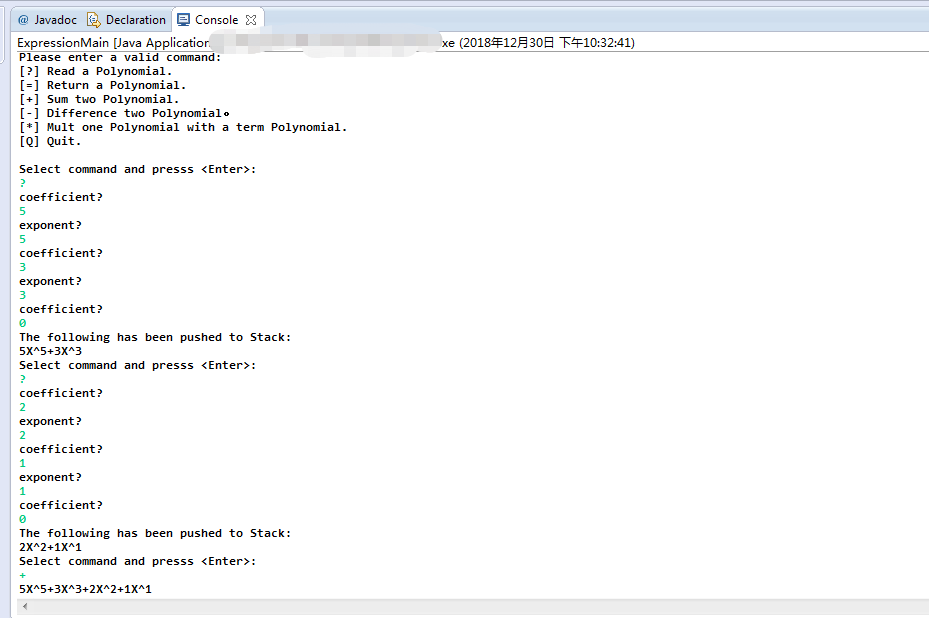展开全文• 最方便，最实用的多项式运算器，方程组求解器，希望对大家有用。
• 本程序为云南大学软件学院数据结构实验，实验要求实现一元稀疏多项式的降幂排列、求导、加减乘；要求实现线性方程组求解。
• 在MFC应用程序中，使用C++语言，编写了可以进行线性方程组求解的简单计算器
• 采用WindowsBuilder构建界面，用HashMap储存多项式，实现多项式的加减运算，求导。通过最简行列式实现对求线性方程组的求解，并输出通解。有较好的容错处理，输入时指数用^表示，下标用_表示，eg：3X^2 2X_1数据结构实验
• ## XDOJ-一元稀疏多项式计算器

千次阅读 热门讨论 2020-12-17 11:21:27
一元稀疏多项式计算器 要变得更强。 这个问题怎么说，一个上午就这么过去了。果然不愧是小白：（ 一开始我还想用三个数组，一个存第一个，一个存第二个，再把结果存到另外一个数组里面。 不过很明显，非常麻烦，当给...

## 一元稀疏多项式计算器

要变得更强。
————更新————
下面同学说的问题我改正啦。
没改之前：可以看到多了个负号，是因为在这个结构体里面，第一项就是0，因此会跳过打印多项式对fooo的求解，所以会多一项负号。
因此我选择用for循环来找到第一项不是0的就可以啦。
改后：——————————
这个问题怎么说，一个上午就这么过去了。果然不愧是小白：（
一开始我还想用三个数组，一个存第一个，一个存第二个，再把结果存到另外一个数组里面。
不过很明显，非常麻烦，当给我把代码码出来时，得到的结果也很离谱。然后……然后我就几乎全部重改了。
然后缩减至两个数组，将无论加减都放在一个数组里。
再将结果放进另外一个数组里。
需要考虑的点：

1.关于系数为0 ，1，-1
2.关于幂次为0，1
3.如果和为0（使用count来计数）


问题描述

一元 n 次多项式𝑝0𝑋𝑒0 + 𝑝1𝑋𝑒1 + ⋯ + 𝑝𝑖𝑋𝑒𝑖 + ⋯ + 𝑝𝑛𝑋𝑒𝑛
项数较少时成为一元稀疏多项式， 例如：3 + 6𝑋3 − 2𝑋8 + 12𝑋20是一个一元稀疏多项式。设计一个一元稀疏多项式计算器程
序完成两个一元稀疏多项式的加减法，输出结果多项式的各项系数和指数。

输入说明

输入数据第 1 行为 3 个正整数 n，m，t。
其中 n 表示第一个多项式的项数，m 表示第二个多项式的项数，t 表示运算类型，0为加法，1 为减法。
数据的第 2 行包含 2n 个整数，每两 个整数分别表示第一个多项式每一项的系数和指数；第 3 行包含 2m 个整数，每两个整数分 别表示第二个多项式每一项的系数和指数。两个多项式的每项是按照指数递增的形式给出的， 例如对于多项式3 + 6𝑋3 − 2𝑋8 + 12𝑋20，对应的输入为 3 0 6 3 -2 8 12 20。

输出说明

运算结果按指数从低到高的顺序在以多项式形式（见输出样例）输出结果，注意系数为负数 时输出减号，系数为 0 时不输出该项，指数为 1
时不输出指数。

输入样例

6 2 0 1 0 1 1 1 2 1 3 1 4 1 5
-1 3 -1 4

输出样例

1+x+x^2 +x^5

我的代码

#include<stdio.h>
typedef struct{
struct{
int ratio,power;
}items;
}POLYNOMIA;

int main(){
POLYNOMIA f,fooo;
int n,m,t,i,j,temp1,temp2,ra,po;
int count=1,k=0,sum=0;

scanf("%d %d %d",&n,&m,&t);//输入数字
//printf("N:%d M:%d T:%d\n",n,m,t);
for(i=0;i<n;i++) scanf("%d %d",&f[i].items.ratio,&f[i].items.power);
for(j=0;j<m;j++,i++) {
scanf("%d %d",&ra,&po);//在输入时就验证是加法还是减法
if(t){//t=1是减法
f[i].items.ratio=-ra;
f[i].items.power=po;
}
else{//加法
f[i].items.ratio=ra;
f[i].items.power=po;
}
}
//进行排序
for(i=0;i<m+n;i++){
for(j=0;j<m+n-i-1;j++){
if(f[j].items.power>f[j+1].items.power){
temp1=f[j].items.power;
f[j].items.power=f[j+1].items.power;
f[j+1].items.power=temp1;

temp2=f[j].items.ratio;
f[j].items.ratio=f[j+1].items.ratio;
f[j+1].items.ratio=temp2;
}
}
}
//进行计算
for(i=0;i<m+n;i++){
if(f[i].items.power!=f[i+1].items.power){
fooo[k].items.power=f[i].items.power;
fooo[k].items.ratio=f[i].items.ratio;
//	printf("fooo%d:%d %d\n",k,fooo[k].items.ratio,fooo[k].items.power);
k++;
}
if(f[i].items.power==f[i+1].items.power){
f[i+1].items.ratio+=f[i].items.ratio;
}
}
//打印多项式
for(i=0;fooo[i].items.ratio==0;i++);
if(fooo[i].items.ratio!=0){//由于第一位不带加减号。
count=0;
if(fooo[i].items.ratio==1){
if(fooo[i].items.power==0)
printf("%d",fooo.items.ratio);
else if(fooo[i].items.power==1)
printf("x");
else
printf("x^%d",fooo[i].items.power);
}
else if(fooo[i].items.ratio==-1){
if(fooo[i].items.power==0)
printf("%d",fooo[i].items.ratio);
else if(fooo[i].items.power==1)
printf("-x");
else
printf("-x^%d",fooo[i].items.power);
}
else{
if(fooo[i].items.power==0)
printf("%d",fooo[i].items.ratio);
else if(fooo[i].items.power==1)
printf("%dx",fooo[i].items.ratio);
else
printf("%dx^%d",fooo[i].items.ratio,fooo[i].items.power);
}
}
for(i++;i<k;i++){//对后面的数字进行加减
if(fooo[i].items.ratio>0){//如果系数是大于0的数字
count=0;
if(fooo[i].items.ratio==1){// 要特别注意1的情况
if(fooo[i].items.power==0)
printf("%d",fooo[i].items.ratio);
else if(fooo[i].items.power==1)
printf("+x");
else
printf("+x^%d",fooo[i].items.power);
}
else{

if(fooo[i].items.power==0)
printf("+%d",fooo[i].items.ratio);
else if(fooo[i].items.power==1)
printf("+%dx",fooo[i].items.ratio);
else
printf("+%dx^%d",fooo[i].items.ratio,fooo[i].items.power);
}
}
else if(fooo[i].items.ratio==0){
count=1;
continue;
}
else{//如果本身是负数，既有符号，就不需要再加上，多余
count=0;
if(fooo[i].items.ratio==-1){

if(fooo[i].items.power==0)
printf("%d",fooo[i].items.ratio);
else if(fooo[i].items.power==1)
printf("-x");
else
printf("-x^%d",fooo[i].items.power);
}
else{
if(fooo[i].items.power==0)
printf("%d",fooo[i].items.ratio);
else if(fooo[i].items.power==1)
printf("%dx",fooo[i].items.ratio);
else
printf("%dx^%d",fooo[i].items.ratio,fooo[i].items.power);
}
}
}
if(count)printf("0");
return 0;
}


130行太多了太多了——
对于一个C的基础题，这就像你写1+1；
写过程用了nnnnnn个方程来解决一样。
哎呀，就是比喻不大确切。
不过，要是能够优化，请扣我！！！
乐意至极，谢谢。

展开全文c语言
• 该项目是本小组团队设计和制作的集线性方程组求解器和一元系数多项式计算为一体的数学工具，主要运用到所学习的数据结构中的链表、结构体、数组思想，并用C#进行编程完成。希望大家参与试用并提出宝贵意见，我们将...
• c++多项式计算器,可进行多项式的加减求导，可以解线性方程组。
• 萌新练手 import math def qua(a, b, c): a1 = -b - math.sqrt(b * b - 4 * a * c) ...a1, b1, c1 = map(int, input("☆一元二次方程计算器☆，请输入a,b,c系数，以空格隔开！\n☆输入0 0 0可退出程序☆\n\n").split()python
• 可以方便地进行矩阵的分解，求解线性方程组，以及进行多项式操作等。在输入矩阵时可 以直接输入数学表达式包括复数，例如可以直接输入sin(i+1)^2，而不同提前计算具体数值 。可以直接输入pi。使用说明在压缩包里。...
• 这是这两天写的第二个项目，准确的来书我还没有完全掌握，很多地方都是借鉴的老师讲的和网上一些代码。 而且这个程序我写了很多头文件和函数文件，还不怎么好发。 我整理了一下，决定就发几个头文件和几个核心函数...
• 科学计算器1、科学计算器程序支持矩阵的加、减、乘、除、求秩、求逆、特征值、广义逆、QR分解、LU分解、Schur分解、奇异值分解、满秩分解、约当标准化、Givens变换、化为Hessenberg矩阵……2、程序支持常规线性方程...
• 主要介绍了Java实现求解一元n次多项式的方法,涉及java高斯消元法处理矩阵运算解多项式的相关操作技巧,需要的朋友可以参考下
• 我们经常听到许多儿童甚至许多成人抱怨他们在解决复杂的多项式方程式时面临的困难程度。 对于许多人来说，遵循科学计算器中的步骤并找到方程式的根源也是困难的。 Therefore, this is a program that would help ...python java 算法 leetcode c++
• 例如，方程 P(x) = x4+ 7x3- 5x + 9 可以表示为： p = [1 7 0 -5 9]； MATLAB计算多项式 MATLAB中 polyval 函数用于将指定的值 - 计算多项式。例如，要计算我们本节开始时举例的多项式 p， x = 4，输入: p...MATLAB
• 日常计算、函数绘图、分式化简、方程组求解、多项式分解和展开、微积分，统统包含其中，比科学计算器还要强大。工作，学习都会方便许多。软件特色1、可以自定进行计算，获得更多的计算服务，为用户提供更多的帮助；2...
• #include #include #include #include using namespace std; double a,b,c; int f; char s; void process(char *p){ int i,z,t=1; double x,k=0; i=0; if(p[i]=='-'){t=-1;i++;} for(z=0;...if(p
• 多项式是数学中常用的一种表达式，现在我们给出用c语言编程实现多项式的计算，并且多项式的计算是链表的典型应用，通过编程实现多项式，也为我们巩固一下链表的知识以及它的生活应用。 下面给出代码 #include <...链表 指针 数据结构 c语言
• 二叉树表达计算器它用二叉树表示任何多项式方程，并通过递归评估左右子树来求解所有运算。TreeWalker.java Treewalker文件包括对树的二叉树状旋转，对树中删除或插入节点，评估，区分和简化所有操作。 方法包括： ...Java
• 代数：多项式，多项式方程组求解，矩阵，分数，衍生工具，复数和更多。显示您键入的结果。使用菜单模式之间进行切换。 Mathlab Graphing Calculator PROMathlab Graphing Calculator PRO 图形计算器 Mathlab ...
• 计算器 矩阵 行列式 微积分 和与积 方程组 统计 表达式 图论 常数 多项式 命题逻辑 川大软件天才 姜边制造
• 费了一番功夫。采取了拆分多项式逐项处理的方法。编写了一个项处理函数，将字符串拆分成一个个项，逐个处理，提取出左式跟右式的系数做差，然后利用求根公式。 Debug记录： ...设计一个二次方程计算器
• 可以解方程组，矩阵行列式，逻辑运算包括计算器，矩阵，行列式，方程多项式等的计算...